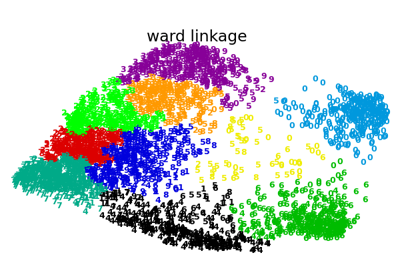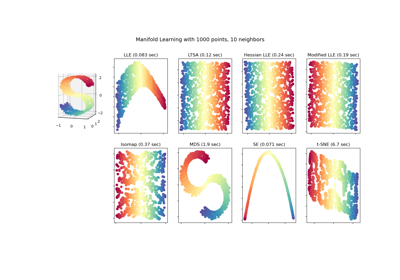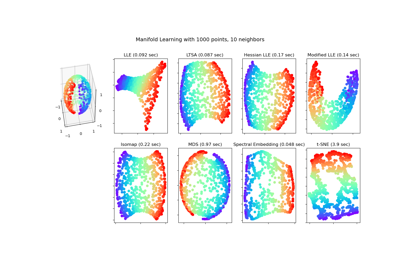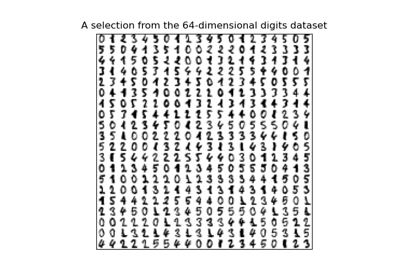# sklearn.manifold.SpectralEmbedding¶

class sklearn.manifold.SpectralEmbedding(n_components=2, *, affinity='nearest_neighbors', gamma=None, random_state=None, eigen_solver=None, n_neighbors=None, n_jobs=None)[source]

Spectral embedding for non-linear dimensionality reduction.

Forms an affinity matrix given by the specified function and applies spectral decomposition to the corresponding graph laplacian. The resulting transformation is given by the value of the eigenvectors for each data point.

Note : Laplacian Eigenmaps is the actual algorithm implemented here.

Read more in the User Guide.

Parameters
n_componentsinteger, default: 2

The dimension of the projected subspace.

affinitystring or callable, default
How to construct the affinity matrix.
• ‘nearest_neighbors’ : construct the affinity matrix by computing a graph of nearest neighbors.

• ‘rbf’ : construct the affinity matrix by computing a radial basis function (RBF) kernel.

• ‘precomputed’ : interpret X as a precomputed affinity matrix.

• ‘precomputed_nearest_neighbors’ : interpret X as a sparse graph of precomputed nearest neighbors, and constructs the affinity matrix by selecting the n_neighbors nearest neighbors.

• callable : use passed in function as affinity the function takes in data matrix (n_samples, n_features) and return affinity matrix (n_samples, n_samples).

gammafloat, optional, default

Kernel coefficient for rbf kernel.

random_stateint, RandomState instance, default=None

Determines the random number generator used for the initialization of the lobpcg eigenvectors when solver == ‘amg’. Pass an int for reproducible results across multiple function calls. See :term: Glossary <random_state>.

eigen_solver{None, ‘arpack’, ‘lobpcg’, or ‘amg’}

The eigenvalue decomposition strategy to use. AMG requires pyamg to be installed. It can be faster on very large, sparse problems.

n_neighborsint, default

Number of nearest neighbors for nearest_neighbors graph building.

n_jobsint or None, optional (default=None)

The number of parallel jobs to run. None means 1 unless in a joblib.parallel_backend context. -1 means using all processors. See Glossary for more details.

Attributes
embedding_array, shape = (n_samples, n_components)

Spectral embedding of the training matrix.

affinity_matrix_array, shape = (n_samples, n_samples)

Affinity_matrix constructed from samples or precomputed.

n_neighbors_int

Number of nearest neighbors effectively used.

References

Examples

>>> from sklearn.datasets import load_digits
>>> from sklearn.manifold import SpectralEmbedding
>>> X.shape
(1797, 64)
>>> embedding = SpectralEmbedding(n_components=2)
>>> X_transformed = embedding.fit_transform(X[:100])
>>> X_transformed.shape
(100, 2)


Methods

 fit(X[, y]) Fit the model from data in X. fit_transform(X[, y]) Fit the model from data in X and transform X. get_params([deep]) Get parameters for this estimator. set_params(**params) Set the parameters of this estimator.
__init__(n_components=2, *, affinity='nearest_neighbors', gamma=None, random_state=None, eigen_solver=None, n_neighbors=None, n_jobs=None)[source]

Initialize self. See help(type(self)) for accurate signature.

fit(X, y=None)[source]

Fit the model from data in X.

Parameters
X{array-like, sparse matrix}, shape (n_samples, n_features)

Training vector, where n_samples is the number of samples and n_features is the number of features.

If affinity is “precomputed” X : {array-like, sparse matrix}, shape (n_samples, n_samples), Interpret X as precomputed adjacency graph computed from samples.

Returns
selfobject

Returns the instance itself.

fit_transform(X, y=None)[source]

Fit the model from data in X and transform X.

Parameters
X{array-like, sparse matrix}, shape (n_samples, n_features)

Training vector, where n_samples is the number of samples and n_features is the number of features.

If affinity is “precomputed” X : {array-like, sparse matrix}, shape (n_samples, n_samples), Interpret X as precomputed adjacency graph computed from samples.

Returns
X_newarray-like, shape (n_samples, n_components)
get_params(deep=True)[source]

Get parameters for this estimator.

Parameters
deepbool, default=True

If True, will return the parameters for this estimator and contained subobjects that are estimators.

Returns
paramsmapping of string to any

Parameter names mapped to their values.

set_params(**params)[source]

Set the parameters of this estimator.

The method works on simple estimators as well as on nested objects (such as pipelines). The latter have parameters of the form <component>__<parameter> so that it’s possible to update each component of a nested object.

Parameters
**paramsdict

Estimator parameters.

Returns
selfobject

Estimator instance.

## Examples using sklearn.manifold.SpectralEmbedding¶